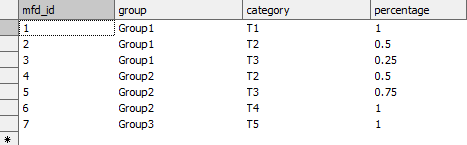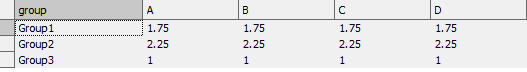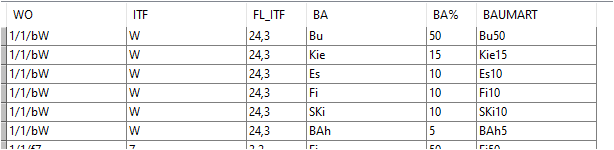Home - General / All posts - Create subgroups using percentages
 Mike Pelletier2,087 post(s) #27-Mar-23 18:20 Struggling to find a good SQL approach to group records into new categories while summing fields but also applying a percentage to values. Normally I'd use Case to do the grouping, but I'm not seeing a way to apply percentages. The attached map file should make this clear with sample data. It would be great to find a slick SQL method because my data set is fairly complicated and the underlying is subject to change.Any thoughts much appreciated.
 sga30 post(s) #28-Mar-23 09:03 Hi mike,I'm not sure I understood your question correctly, so please tell me if I don't answer it. Take a look at the result table (second picture) to see if that's the result you're looking for.I propose to create a table that lists the group and states the percentage of each category (one line per unique combination of group and category). Something like this in your datasetThen join your newly created table with your test table. Group by [group] and sum your columns multiplied by the percentageSELECT [group], Sum([A]*[percentage]) as [A],Sum([B]*[percentage]) as [B],Sum([C]*[percentage]) as [C],Sum([D]*[percentage]) as [D]FROM [test]INNER JOIN [Table]ON [test].[name]=[Table].[category]GROUP BY [group]This will result inAgain, I hope this is what you were seeking for.See attached file.
 Mike Pelletier2,087 post(s) #28-Mar-23 15:05 That's an excellent solution. Thank you very much!
 Sloots642 post(s) #28-Mar-23 17:48 With the use of the VALUES statement you can omit the intermediate table.SELECT [group],  Sum([A]*[percentage]) as [A] , Sum([B]*[percentage]) as [B] , Sum([C]*[percentage]) as [C] , Sum([D]*[percentage]) as [D]FROM [test]INNER JOIN ( VALUES  ('Group 1', 'T1', 1) , ('Group 1', 'T2', 0.5) , ('Group 1', 'T3', 0.25) , ('Group 2', 'T2', 0.5) , ('Group 2', 'T3', 0.75) , ('Group 2', 'T4', 1) , ('Group 3', 'T5', 1)  AS ([group], [category], [percentage]) )ON [test].[name] = [category]GROUP BY  [group] http://www.mppng.nl/manifold/pointlabeler
 Mike Pelletier2,087 post(s) #28-Mar-23 19:04 Thanks Chris! Embedding the table in the query. Slick.
 volker1,082 post(s) #05-Apr-23 05:48 Hello,someone an idea how to group something like this:after grouping it should looks like this:1/1/bW -- BU50 Kie15 Es10 Fi10 SKi10 BAh5Thanks for advance http://www.thegisservicesector.de
 Dimitri7,233 post(s) #05-Apr-23 06:44 Use the StringJoinTokens aggregate function together with COLLECT DISTINCT.If you use the forum search box to search on StringJoinTokens you get useful hits like this one.There are also related topics in the user manual like this one.
 volker1,082 post(s) #05-Apr-23 07:43 Thank you Dimitrihttp://www.thegisservicesector.de